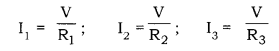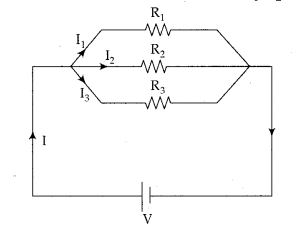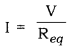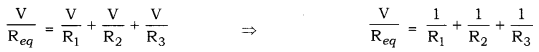# Mention how you will connect the ammeter and the voltmeter

Explain with the help of a labelled circuit diagram how you will find the resistance of a combination of three resistors, or resistance R1, R2, R3, joined in parallel. Also mention how you will connect the ammeter and the voltmeter in the circuit when measuring the current in the circuit and the potential difference across one of the three resistors of the combination.

The given figure shows a circuit consisting of three resistors R1, R2 and R3 connected in parallel. The total current in the circuit (I) gets divided among the three resistors as I1, I2 and I3. Thus,
I = I1 = I2 = I3
Applying Ohm’s law to each resistor,Let the equivalent resistance of the circuit be R Applying Ohm’s law to the equivalent circuitUsing this,An ammeter has to be connected in series with the combination of all three resistors so that, the current passes through the ammeter becomes equal to the total current through the circuit.

The voltmeter has to be connected in parallel to that resistor across which, the potential difference has to be measured.